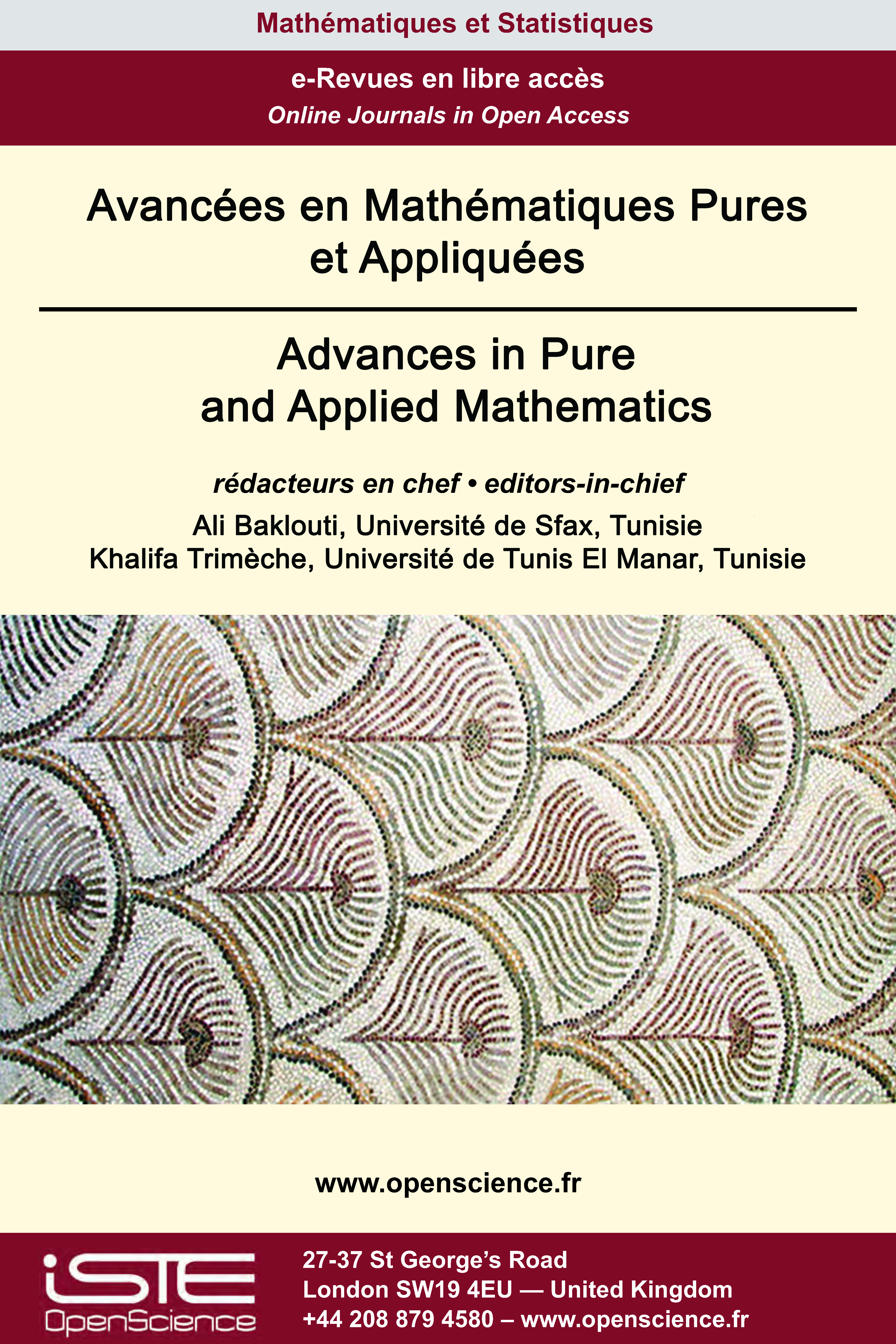# Vol 12 - Issue 2 (May 2021)

## List of Articles

Approximation of Complex-Valued Functions by Fractal Functions

Fractal approximants developed through iterated function systems (IFS) prove more versatile than classical approximants. In this paper, we introduce a new class of fractal approximants using the suitable bounded linear operators defined on the space C(I) of continuous functions and concept of $\alpha$-fractal functions. The convergence of the proposed fractal approximants towards the original continuous function does not need any condition on the scaling factors. The fractal approximants proposed in this paper possess fractality and convergence simultaneously. Without imposing any condition on the scaling vector, we establish the constrained approximation by the proposed fractal approximants. Existence of Schauder basis of fractal polynomials for the space of continuous functions C(I) is investigated. Using the proposed class of fractal approximants, we develop complex fractal approximants for representation of the square integrable complex-valued functions defined on a real compact interval.

A priori estimates for super-linear elliptic equation: the Neumann boundary value problem

$\mbox{In this paper we study the nonexistence of finite Morse index solutions of the following} \\\mbox{Neumann boundary value problems}\\ {(Eq.H)} \begin{cases} -\Delta u = (u^{+})^{p} \;\; \text{in  \mathbb{R}_+^N}, \\ \frac{\partial u}{\partial x_{N}}=0 \quad\quad\;\; \text{ on  \partial\mathbb{R}_+^N}, \\ u \in C^2(\overline{\mathbb{R}_+^N}) \mbox{ and sign-changing, }\\u^+ \mbox{ is bounded and } i(u)<\infty,\end{cases}\\ \mbox{or}\\ {(Eq.H')}\begin{cases}-\Delta u = |u|^{p-1}u \;\; \text{in  \mathbb{R}_+^N}, \\ \frac{\partial u}{\partial x_{N}}=0 \;\;\;\;\;\;\;\;\quad\text{ on  \partial\mathbb{R}_+^N}, \\ u \in C^2(\overline{\mathbb{R}_+^N}),\\ u \mbox{ is bounded and } i(u) < \infty.\end{cases}\\ \mbox{ As a consequence, we establish the relevant Bahri-Lions's }L^\infty\mbox{-estimate  via}\\ \mbox{the boundedness of Morse index of solutions to}\\ \begin{equation}\label{1.1} \left\{\begin{array}{lll} -\Delta u=f(x,u) &\text{in  \Omega,}\\ \frac{\partial u}{\partial \nu}=0 &\text{on \partial \Omega,} \end{array} \right. \end{equation}\\ \mbox{where} f \mbox{ has an asymptotical behavior at in-nity} \mbox{which is not necessarily the same at} \pm\infty. \\\mbox{Our results complete previous Liouville type theorems and } L^\infty\mbox{-bounds via Morse index} \\\mbox{ obtained in [3, 6, 13, 16, 12, 21].}$

Maximal operator in Dunkl-Fofana spaces

We generalize Wiener amalgam spaces by using Dunkl translation instead of the classical one, and we give some relationship between these spaces, Dunkl-Lebesgue spaces and Dunkl-Morrey spaces. We prove that the Hardy-Litlewood maximal function associated with the Dunkl operators is bounded on these generalized Dunkl-Morrey spaces.

On the control of a nonlinear system of viscoelastic equations

In this paper we consider a nonlinear system of two coupled viscoelastic equations, prove the well posedness, and investigate the asymptotic behaviour of this system. We use minimal and general conditions on the relaxation functions and establish explicit energy decay formula which gives the best decay rates expected under this level of generality. Our new result generalizes the earlier related results in the literature.### Other issues :

2020

Volume 20- 11

Issue 1 (May 2020)
Issue 2 (September 2020)

Forthcoming papers

2021

Volume 21- 12

Issue 1 (January 2021)
Issue 2 (May 2021)